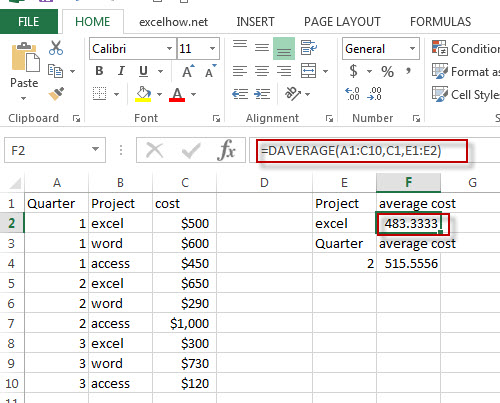# Excel Daverage Function

This post will guide you how to use Excel DAVERAGE function with syntax and examples in Microsoft excel.

### Description

The Excel DAVERAGE function calculates the average of values in a column of a list or database that match conditions you specify.

The DAVERAGE function is a build-in function in Microsoft Excel and it is categorized as a Database Function.

The DAVERAGE function is available in Excel 2016, Excel 2013, Excel 2010, Excel 2007, Excel 2003, Excel XP, Excel 2000, Excel 2011 for Mac.

### Syntax

The syntax of the DAVERAGE function is as below:

`= DAVERAGE(database, field, criteria)`

Where the DAVERAGE function arguments are:

• Database -This is a required argument.  The range of cells that containing the database.
• Field – This is a required argument.  The column to average the values.
• Criteria – The range of cells that contains the conditions that you specify.

### Excel DAVERAGE Function Examples

The below examples will show you how to use Excel DAVERAGE Function to average all numbers in a column of a list or database based on a given criteria.

#1 =DAVERAGE(A1:C10,C1,E1:E2)Note: The above formula will return the average cost of project excel in the three quarter.